alexkras.com Go back Open original

# Reverse Engineering One Line of JavaScript

By Alex Kras

Few months ago I saw an email asking if someone could unwrap this one line of JavaScript.

``````<pre id=p><script>n=setInterval("for(n+=7,i=k,P='p.\\n';i-=1/k;P+=P[i%2?(i%2*j-j+n/k^j)&1:2])j=k/i;p.innerHTML=P",k=64)</script>
``````

This line will render just like the image below. You can see it in your browser here. It was created by Mathieu ‘p01’ Henri, author of www.p01.org where you can find this and many other cool demos.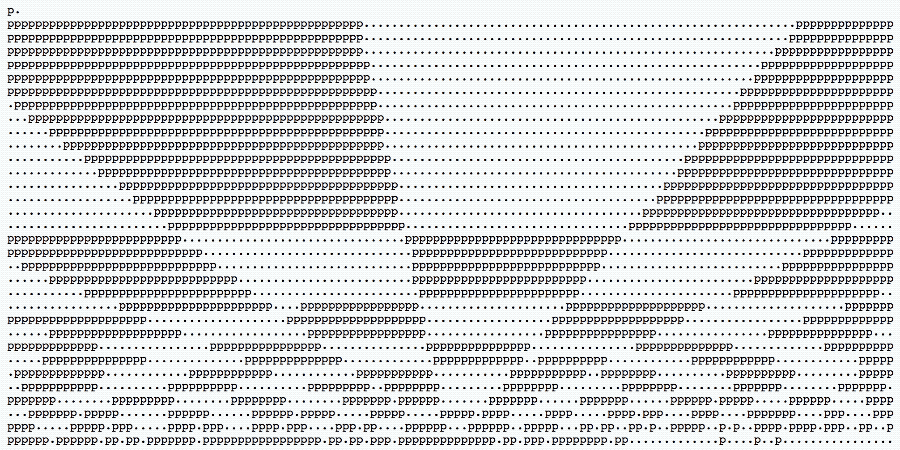Challenge accepted!

## Part 1. Making the code readable

First things first, I kept HTML in the HTML and moved JavaScript into a `code.js` file. I also wrapped p in the `id="p"` in quotes.

index.html

``````<script src="code.js"></script>
<pre id="p"></pre>
``````

I noticed that there was a variable `k`, which was just a constant, so I moved it out of the line, and renamed it as `delay`.

code.js

``````var delay = 64;
var draw = "for(n+=7,i=delay,P='p.\\n';i-=1/delay;P+=P[i%2?(i%2*j-j+n/delay^j)&1:2])j=delay/i;p.innerHTML=P";
var n = setInterval(draw, delay);
``````

Next, the `var draw` was just a string, that was being executed as `eval` within setInterval, since setInterval can accept a function or a string to be evaluated. I moved it to an actual function. I kept the old line there, though, for reference.

Another thing that I noticed was that element `p` was actually referring to the DOM element with id `p` that was declared in HTML, the one I recently wrapped in quotes. Turns out elements can be referenced by their id name from JavaScript, as long as the id name is made up of alpha-numeric characters only. I added the `document.getElementById("p")` to make it more intuitive.

``````var delay = 64;
var p = document.getElementById("p"); // < --------------
// var draw = "for(n+=7,i=delay,P='p.\\n';i-=1/delay;P+=P[i%2?(i%2*j-j+n/delay^j)&1:2])j=delay/i;p.innerHTML=P";
var draw = function() { for (n += 7, i = delay, P = 'p.\n'; i -= 1 / delay; P += P[i % 2 ? (i % 2 * j - j + n / delay ^ j) & 1 : 2]) { j = delay / i; p.innerHTML = P; }
};
var n = setInterval(draw, delay);
``````

Next, I declare the variables `i`, `p`, and `j`, and moved them to the top of the function.

``````var delay = 64;
var p = document.getElementById("p");
// var draw = "for(n+=7,i=delay,P='p.\\n';i-=1/delay;P+=P[i%2?(i%2*j-j+n/delay^j)&1:2])j=delay/i;p.innerHTML=P";
var draw = function() { var i = delay; // < --------------- var P ='p.\n'; var j; for (n += 7; i > 0 ;P += P[i % 2 ? (i % 2 * j - j + n / delay ^ j) & 1 : 2]) { j = delay / i; p.innerHTML = P; i -= 1 / delay; }
};
var n = setInterval(draw, delay);
``````

I factored out `for` loop into a `while` loop. Keeping only the CHECK_EVERY_LOOP part of `for`‘s 3 parts: (RUNS_ONCE_ON_INIT; CHECK_EVERY_LOOP; DO_EVERY_LOOP), and moving everything else in or outside of the body of the loop.

``````var delay = 64;
var p = document.getElementById("p");
// var draw = "for(n+=7,i=delay,P='p.\\n';i-=1/delay;P+=P[i%2?(i%2*j-j+n/delay^j)&1:2])j=delay/i;p.innerHTML=P";
var draw = function() { var i = delay; var P ='p.\n'; var j; n += 7; while (i > 0) { // <---------------------- //Update HTML p.innerHTML = P; j = delay / i; i -= 1 / delay; P += P[i % 2 ? (i % 2 * j - j + n / delay ^ j) & 1 : 2]; }
};
var n = setInterval(draw, delay);
``````

I unroll ternary operator ( `condition ? do if true : do if false`) in `P += P[i % 2 ? (i % 2 * j - j + n / delay ^ j) & 1 : 2];`.

`i%2` was checking if `i` was even or odd. If i was even, it was just returning 2. If i was odd, it was returning the magic value of `(i % 2 * j - j + n / delay ^ j) & 1;` (more on that in a bit).

Finally that index was used to offset into string P, so it became `P += P[index];`.

``````var delay = 64;
var p = document.getElementById("p");
// var draw = "for(n+=7,i=delay,P='p.\\n';i-=1/delay;P+=P[i%2?(i%2*j-j+n/delay^j)&1:2])j=delay/i;p.innerHTML=P";
var draw = function() { var i = delay; var P ='p.\n'; var j; n += 7; while (i > 0) { //Update HTML p.innerHTML = P; j = delay / i; i -= 1 / delay; let index; let iIsOdd = (i % 2 != 0); // <--------------- if (iIsOdd) { // <--------------- index = (i % 2 * j - j + n / delay ^ j) & 1; } else { index = 2; } P += P[index]; }
};
var n = setInterval(draw, delay);
``````

I factored out `& 1;` in `index = (i % 2 * j - j + n / delay ^ j) & 1` into another if statement.

This one is a clever way of checking if the result in parentheses is odd or even, and returning 0 for even and 1 for odd. `&` is a bitwise AND operator. Logic for AND is as follows:

Hence `something & 1` will convert “something” to a binary representation, it will also pad 1 with as many 0 in the front as needed, to match the length of something, and will return just an AND of the last bit. For example, 5 in binary is `101`, if we AND it with `1` will get the following:

`````` 101
AND 001 001
``````

In other words, 5 is odd, and result of 5 & 1 is 1. It’s easy to confirm that this logic holds in a JavaScript console.

``````0 & 1 // 0 - even return 0
1 & 1 // 1 - odd return 1
2 & 1 // 0 - even return 0
3 & 1 // 1 - odd return 1
4 & 1 // 0 - even return 0
5 & 1 // 1 - odd return 1
``````

Note that I also renamed rest of `index` to `magic`, so the code with unrolled `&1` will looks as follows.

``````var delay = 64;
var p = document.getElementById("p");
// var draw = "for(n+=7,i=delay,P='p.\\n';i-=1/delay;P+=P[i%2?(i%2*j-j+n/delay^j)&1:2])j=delay/i;p.innerHTML=P";
var draw = function() { var i = delay; var P ='p.\n'; var j; n += 7; while (i > 0) { //Update HTML p.innerHTML = P; j = delay / i; i -= 1 / delay; let index; let iIsOdd = (i % 2 != 0); if (iIsOdd) { let magic = (i % 2 * j - j + n / delay ^ j); let magicIsOdd = (magic % 2 != 0); // &1 < -------------------------- if (magicIsOdd) { // &1 <-------------------------- index = 1; } else { index = 0; } } else { index = 2; } P += P[index]; }
};
var n = setInterval(draw, delay);
``````

Next I unrolled `P += P[index];` into a switch statement. By now it’s clear that index can only be one of three values – 0, 1, or 2. It’s also clear that P always gets initialized with the following values – `var P ='p.\n';`. Where 0 points to `p`, 1 points to `.` and 2 points to `\n` – a new line character.

``````var delay = 64;
var p = document.getElementById("p");
// var draw = "for(n+=7,i=delay,P='p.\\n';i-=1/delay;P+=P[i%2?(i%2*j-j+n/delay^j)&1:2])j=delay/i;p.innerHTML=P";
var draw = function() { var i = delay; var P ='p.\n'; var j; n += 7; while (i > 0) { //Update HTML p.innerHTML = P; j = delay / i; i -= 1 / delay; let index; let iIsOdd = (i % 2 != 0); if (iIsOdd) { let magic = (i % 2 * j - j + n / delay ^ j); let magicIsOdd = (magic % 2 != 0); // &1 if (magicIsOdd) { // &1 index = 1; } else { index = 0; } } else { index = 2; } switch (index) { // P += P[index]; <----------------------- case 0: P += "p"; // aka P break; case 1: P += "."; // aka P break; case 2: P += "\n"; // aka P } }
}; var n = setInterval(draw, delay);
``````

I cleaned up the `var n = setInterval(draw, delay);` magic. Set interval returns an integer starting with 1, and increments it by one every time `setInterval` is called. That integer can be used to `clearInterval` (cancel it). In our case, setInterval was being called just one time, and n was simply getting set to 1.

I also rename `delay` to `DELAY` to remind that it was just a constant.

Last but not least, I put parentheses in `i % 2 * j - j + n / DELAY ^ j` to point out that `^` bitwise XOR has lower precedence then `%`, `*`, `-`, `+`, and `/` operators. In other words all of the computations above will be conducted first, before `^` is evaluated. Resulting in `(i % 2 * j - j + n / DELAY) ^ j)`.

Update: It was pointed out to me, that I mistakenly placed `p.innerHTML = P; //Update HTML` inside the while loop, so I moved it out.

``````const DELAY = 64; // approximately 15 frames per second 15 frames per second * 64 seconds = 960 frames
var n = 1;
var p = document.getElementById("p");
// var draw = "for(n+=7,i=delay,P='p.\\n';i-=1/delay;P+=P[i%2?(i%2*j-j+n/delay^j)&1:2])j=delay/i;p.innerHTML=P"; /** * Draws a picture * 128 chars by 32 chars = total 4096 chars */
var draw = function() { var i = DELAY; // 64 var P ='p.\n'; // First line, reference for chars to use var j; n += 7; while (i > 0) { j = DELAY / i; i -= 1 / DELAY; let index; let iIsOdd = (i % 2 != 0); if (iIsOdd) { let magic = ((i % 2 * j - j + n / DELAY) ^ j); // < ------------------ let magicIsOdd = (magic % 2 != 0); // &1 if (magicIsOdd) { // &1 index = 1; } else { index = 0; } } else { index = 2; } switch (index) { // P += P[index]; case 0: P += "p"; // aka P break; case 1: P += "."; // aka P break; case 2: P += "\n"; // aka P } } //Update HTML p.innerHTML = P;
}; setInterval(draw, 64);
``````

You can see the final result in action here.

## Part 2. Understanding what the code does

So what is going on here? Let’s see.

Initial value of `i` is set to 64 via `var i = DELAY;`, and it’s decremented by 1/64 (0.015625) on every loop via `i -= 1 / DELAY;`. The loop goes on until `i` is no longer greater than 0 `while (i > 0) {`. Every run of the loop, `i` is decremented by 1/64th, so it will take 64 loops for `i` to decrement by 1 (64/64 = 1). In total then `i` would have to be decremented 64 x 64 = 4096 times, to become below 0.

The image consist of 32 rows, with 128 chars in every row. Conveniently 64 x 64 = 32 x 128 = 4096. `i` will only be even (not odd `let iIsOdd = (i % 2 != 0);`) when `i` is strictly an even number. We’ll get it to happen 32 times, when i is 64, 62, 60 etc. These 32 times, the index will be set to 2 `index = 2;` and a new line character will be added to the line `P += "\n"; // aka P`. The rest 127 character per row will either be set to `p` or `.`.

But when do we set `p` and when do we set `.`?

Well, for starters we know that we will set it to `.` when magic `let magic = ((i % 2 * j - j + n / DELAY) ^ j);` is odd, and we’ll set it to `p` when magic is even.

``````var P ='p.\n'; ... if (magicIsOdd) { // &1 index = 1; // second char in P - .
} else { index = 0; // first char in P - p
}
``````

But when is magic odd and when is it even? That’s a million dollar question. Before we talk about that though, let’s establish one more thing.

If we remove `+ n/DELAY` from `let magic = ((i % 2 * j - j + n / DELAY) ^ j);`, we’ll end up with the following static layout, that will not be moving at all.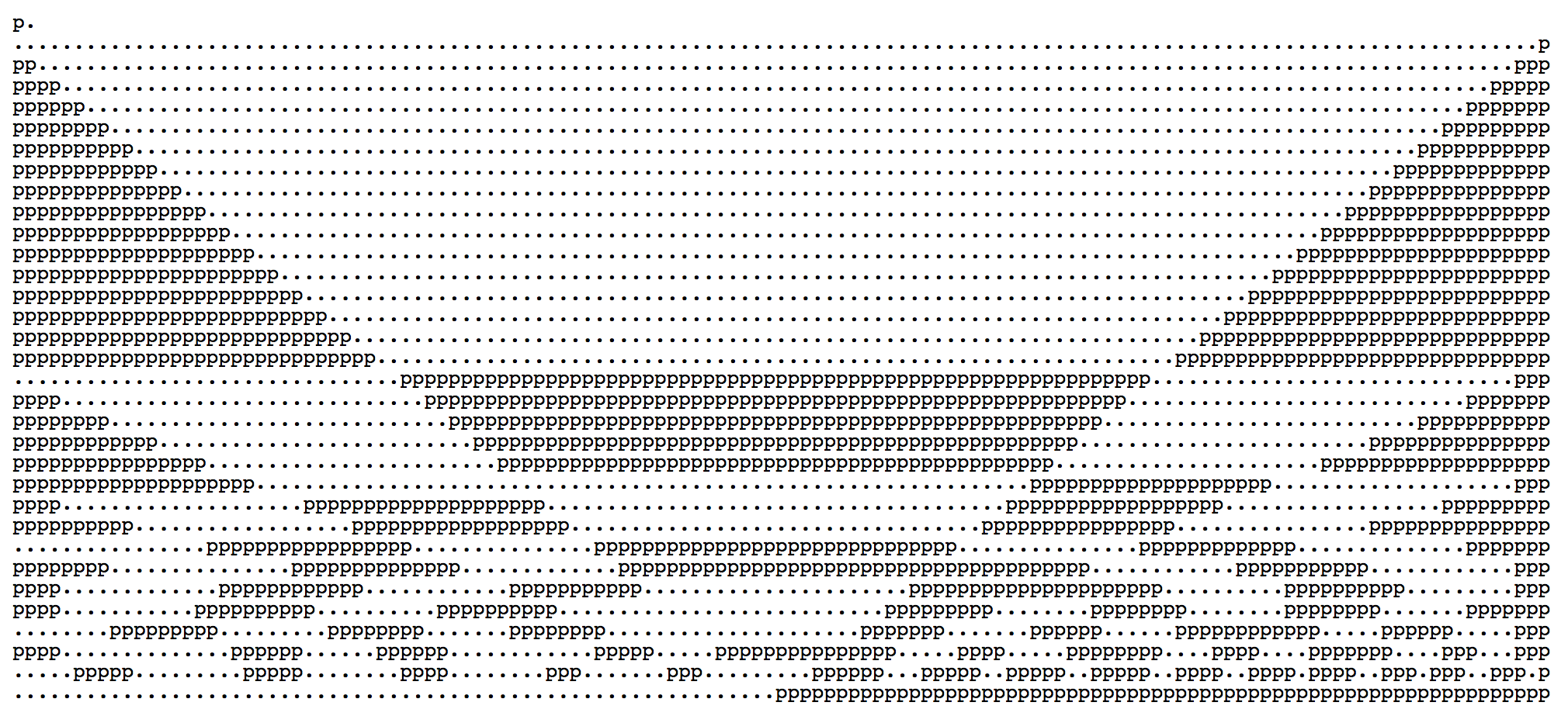For now let’s look at the `magic` with `+ n/DELAY` removed. How do we end up with the pretty picture above?

`(i % 2 * j - j) ^ j`

Note that for every loop we have:

``````j = DELAY / i;
i -= 1 / DELAY;
``````

In other words we can express `j` in terms of the final `i` as `j = DELAY/ (i + 1/DELAY)` but since 1/DELAY is such a small number, for illustration purposed we can drop `+ 1/DELAY` and simplify it to `j = DELAY/i = 64/i`.

Given that we can rewrite `(i % 2 * j - j) ^ j` as `(i % 2 * 64/i - 64/i) ^ 64/i`.

Let’s use an online graphing calculator to plot some of these functions.

First of all, let’s plot `i%2`.

This results in a nice graph, with value of y ranging form 0 to 2.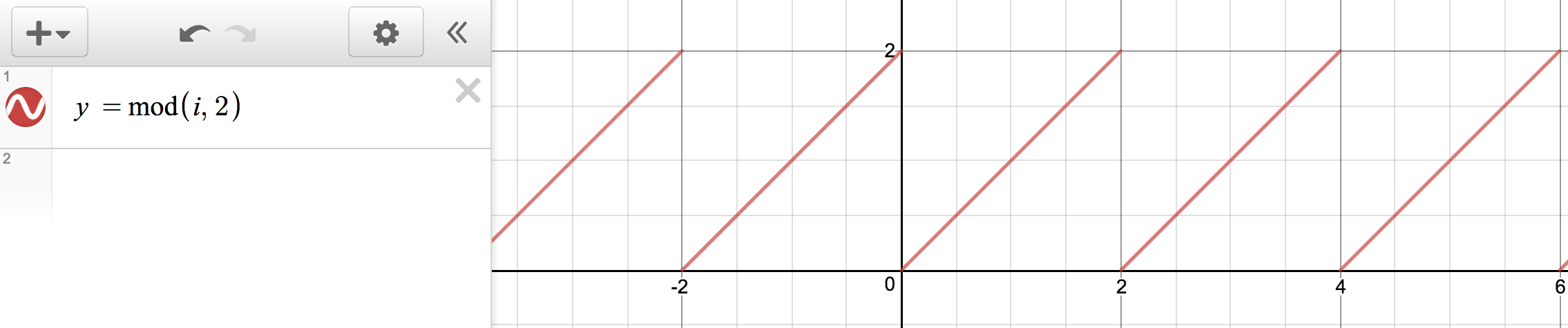If we plot `64/i` will get a graph that looks like this.If we plot the full left side, we’ll get a graph that looks kind of like combination of the 2.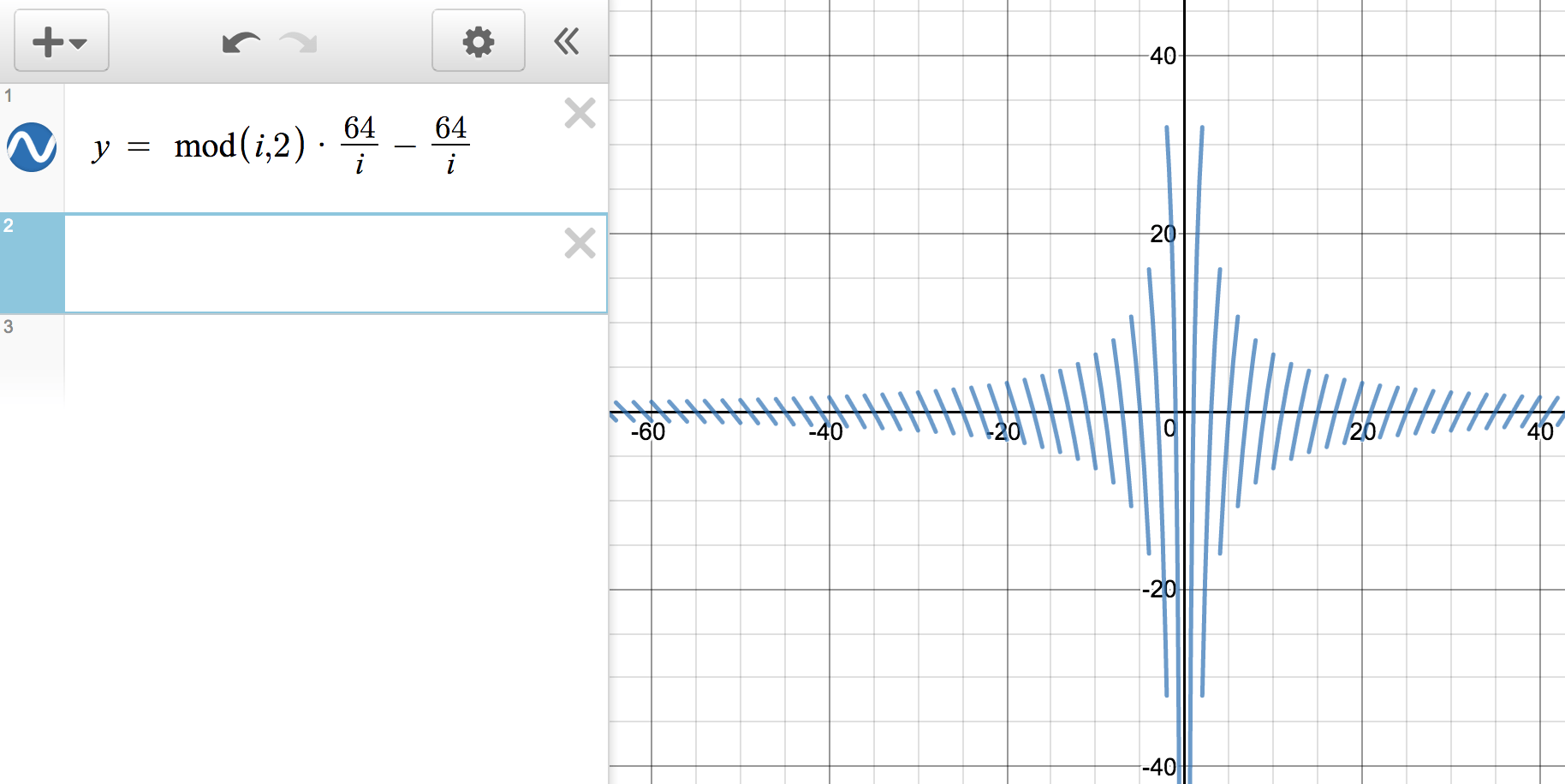Finally, if we plot 2 functions side by side we get the following.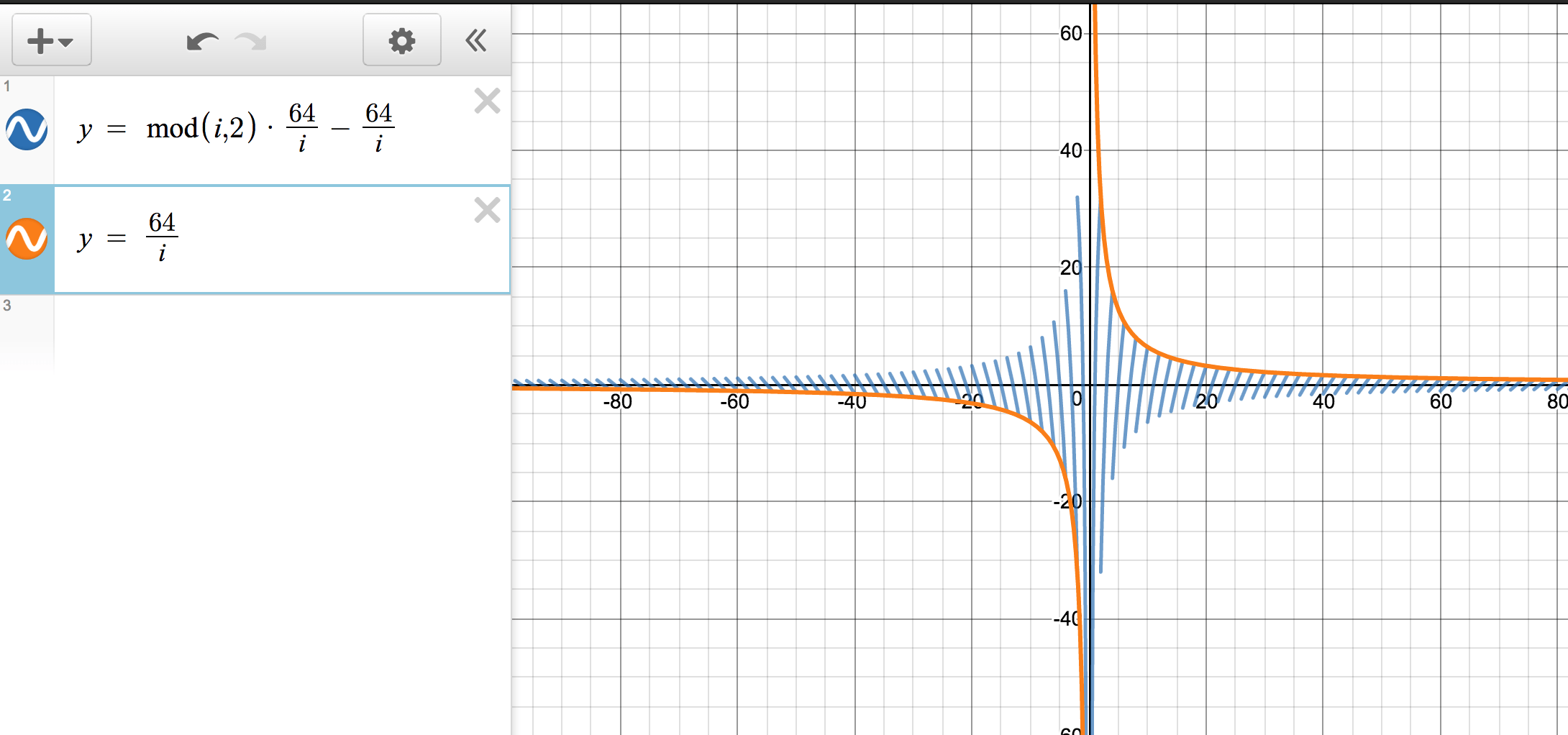### What do all these graphs tell us?

Let’s remind ourselves what question we are trying to answer, and that is how did we ended with a pretty static image like this:Well, we know that if the magic `(i % 2 * j - j) ^ j` results in an even number we’ll have to add `p` and for an odd value number we’ll have to add `.`.

Let’s zoom in on the first 16 rows of our chart, with `i` values ranging from 64 to 32.Bitwise XOR in JavaScript will drop all the values to the right of decimal point, so it’s kind of like taking a `Math.floor` of a number.

It will return 0 when both bits are 1s or both bits are 0s.

Our `j` will start as 1 and will slowly make its way to 2, staying right below it, so we can treat it as 1 (`Math.floor(1.9999) === 1`), and we’ll need another 1 on the left side, to get the result to be 0 (meaning even), giving us a `p`.

In other words, each diagonal green line represents one row in our chart. Since j is always above 1 but below 2 for the first 16 rows, the only way we can get odd value is if left side of `(i % 2 * j - j) ^ j` aka `i % 2 * i/64 - i/64` aka diagonal green line is also above 1 or below -1.

Here are some output from JavaScript console to drive this point home, 0 or -2 means result is even, 1 means result is odd.

``````1 ^ 1 // 0 - even p
1.1 ^ 1.1 // 0 - even p
0.9 ^ 1 // 1 - odd .
0 ^ 1 // 1 - odd .
-1 ^ 1 // -2 - even p
-1.1 ^ 1.1 // -2 - even p
``````

If we look at our graph, we’ll see that the right most diagonal line barely goes above 1 or below -1 (few evens – few `p`s), the next line goes a bit more, and the one after that even more etc. Line number 16 barely stays under 2 and above – 2. After line 16 we can see our static chart shifts a pattern.After line 16 `j` crosses the line of 2, and the expected result flips. Now we’ll get an even number when our green diagonal line is above 2, below -2, or within but not equal to 1 and -1. That is why we see two or more groups of `p`s from line 17 onward.

If you look closely at the bottom few lines of the moving image, you’ll notice that they no longer follow the same pattern, due to wide fluctuation of the graph.

Let’s comeback to `+ n/DELAY` now. In code we can see that n starts out at 8 (1 from setInteval plust 7 on every interval being called). It is then incremented by 7 every time set interval fires.

When n becomes 64, the graph changes to look as follows.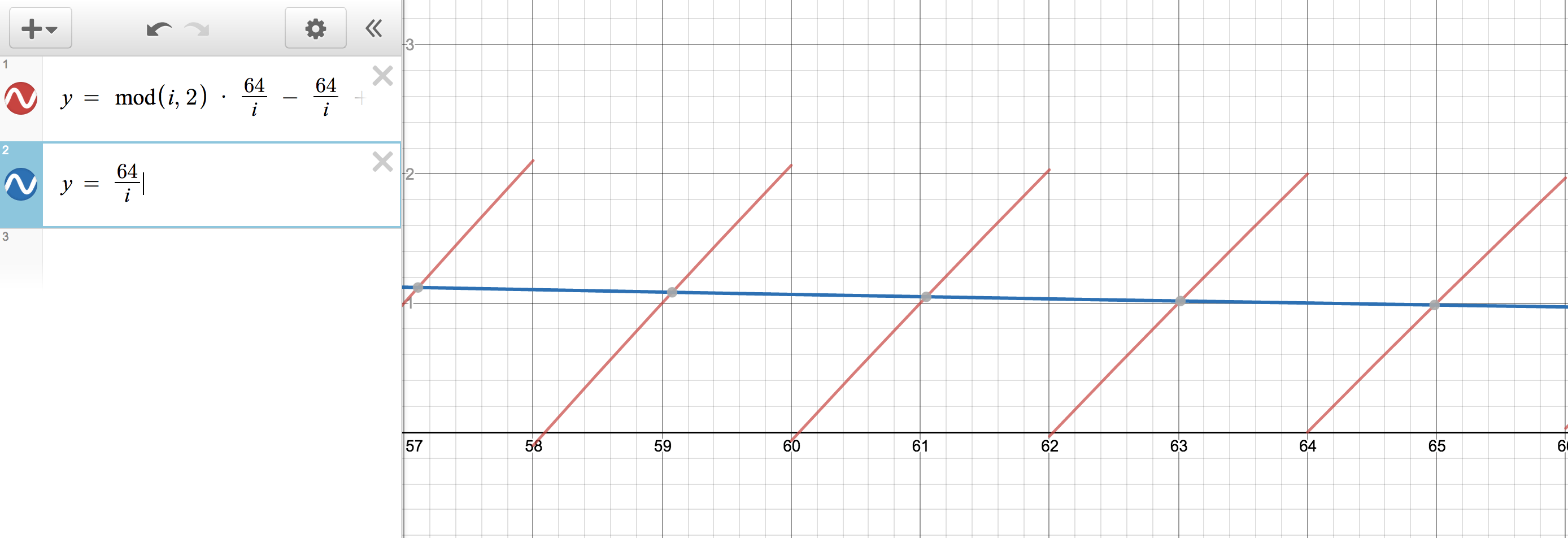Note that `j` is still ~1 (~ here means approximately), but now left half of red diagonal line around 62-63 is ~0 and right half around 63-64 is ~1. Since our chars get populated in decreasing order from 64 to 62, we would expect the 63-64 side of the diagonal line (1 ^ 1 = 0 // even) to append bunch of `p` and left 62-63 side to append bunch of (1^0 = 1 // odd) `.`, that would be growing left to right as normal English words.

Rendered HTML for that condition will look as follows ( you can hard code value for `n` in codepen to see for yourself). Which in fact matches our expectations.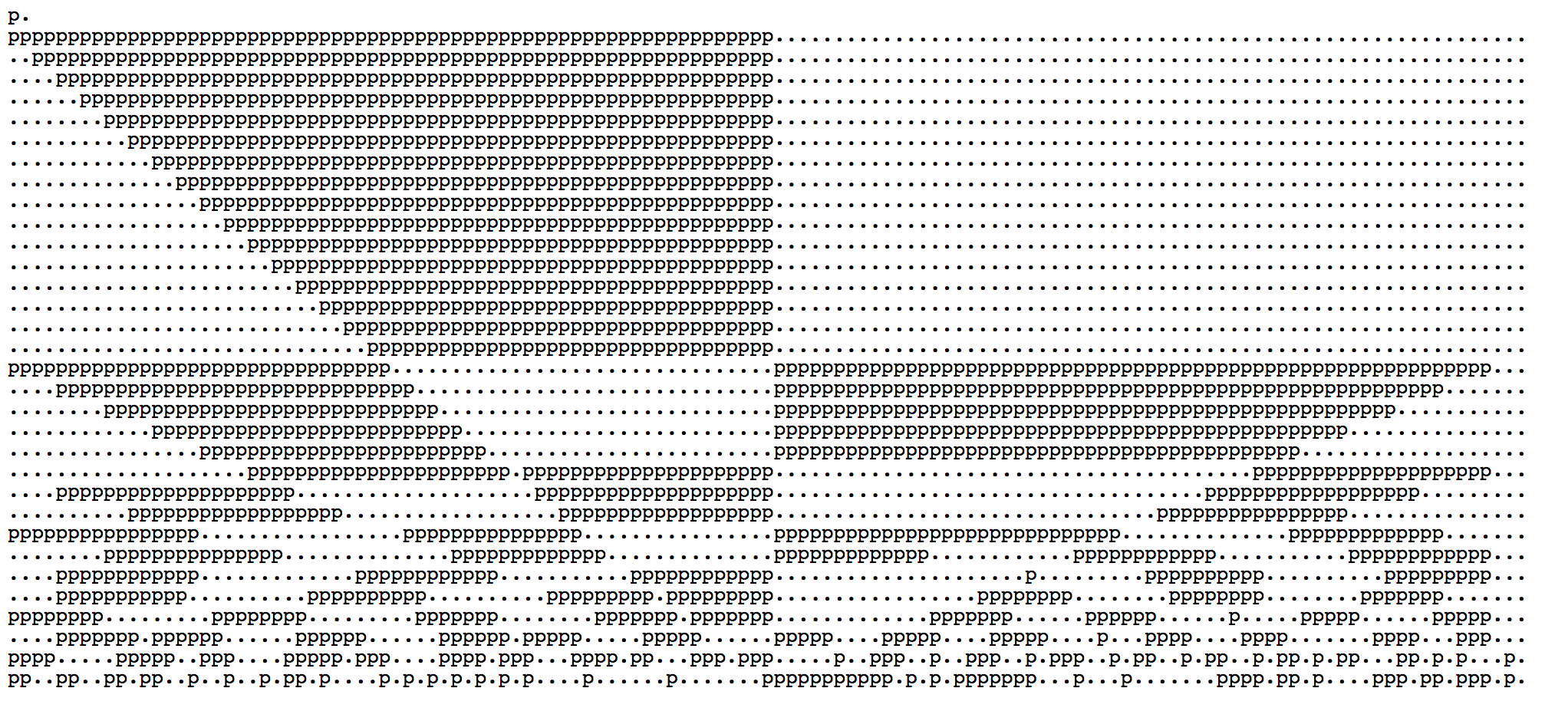At this point number of `p`s has grown to its constant value. For example, for the first row half of all values will always be even. From now on `p`s and `.`s will only shift their position.

To illustrate, when n grows by 7 on the next setInterval, the graph will change slightly.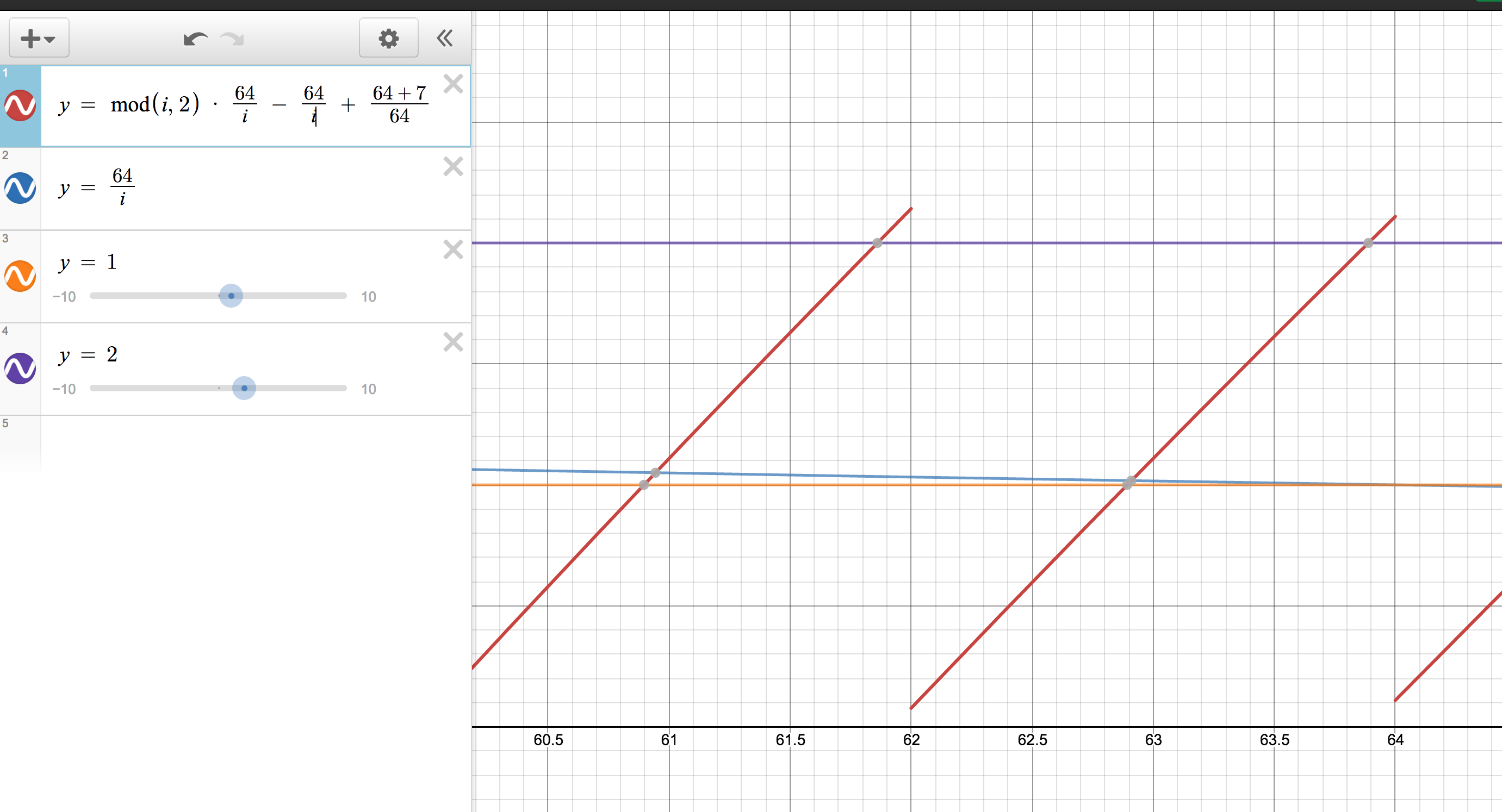Note that diagonal line for the first row (around 64 mark) has move roughly 1 small square up. Assuming that 4 larger square represent 128 chars, 1 large square would represent 32 chars, and 1 small square would represent 32/5 = 6.4 chars (an approximation). If we look at the rendered HTML, we’ll see that first row did in fact move right by 7 chars.One last example. Here is what happens when setInterval gets called 7 more times with n now equal 64+9×7.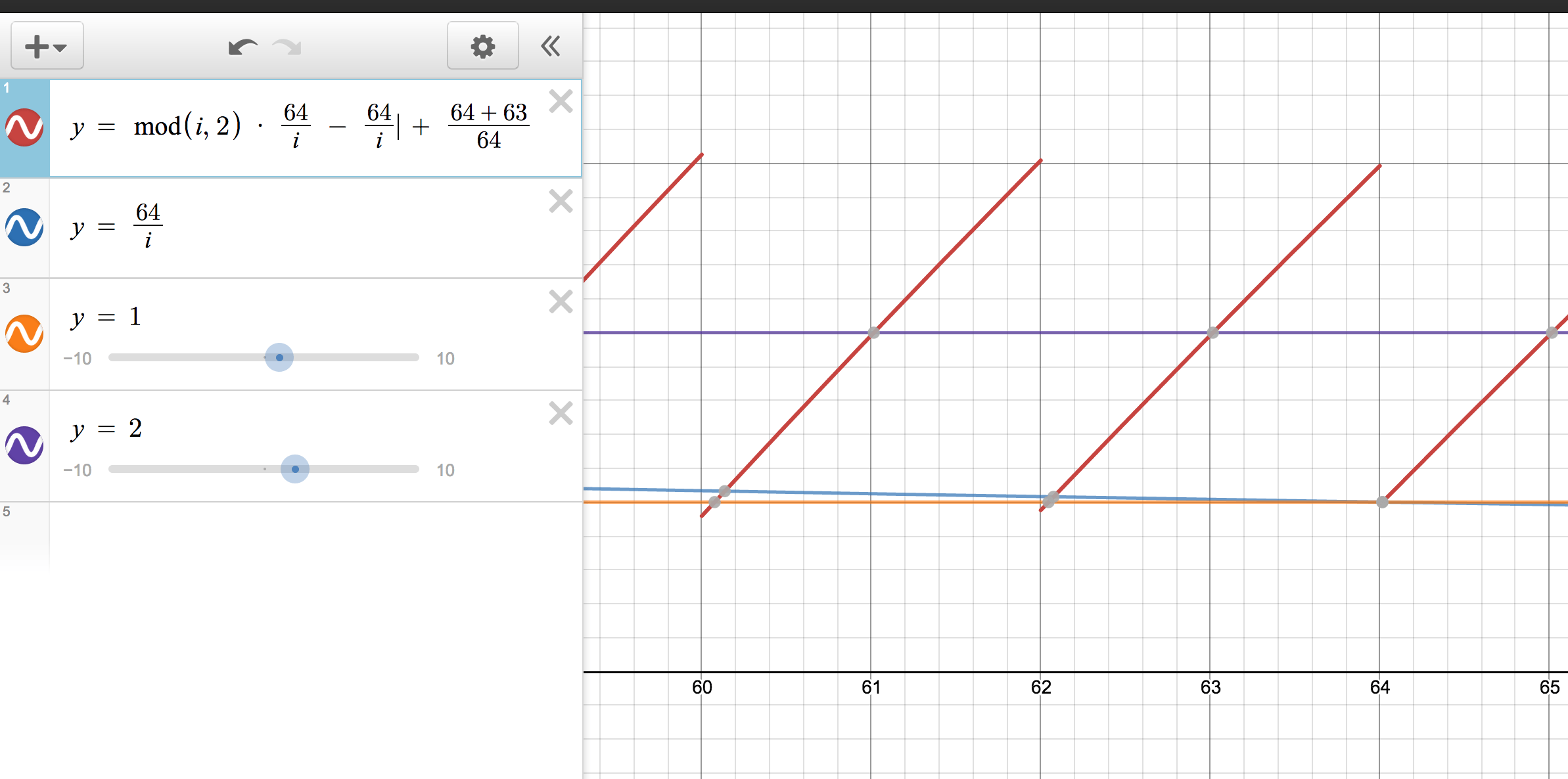For 1st row `j` is still equal to 1. Now the top half of red diagonal line around 64 is ~2, and the bottom one ~1. This flips the picture, since now
`1^2 = 3 // odd - .` and `1 ^ 1 = 0 //even - p`. So we would expect bunch of dots, followed by `p`s.

Which renders as such.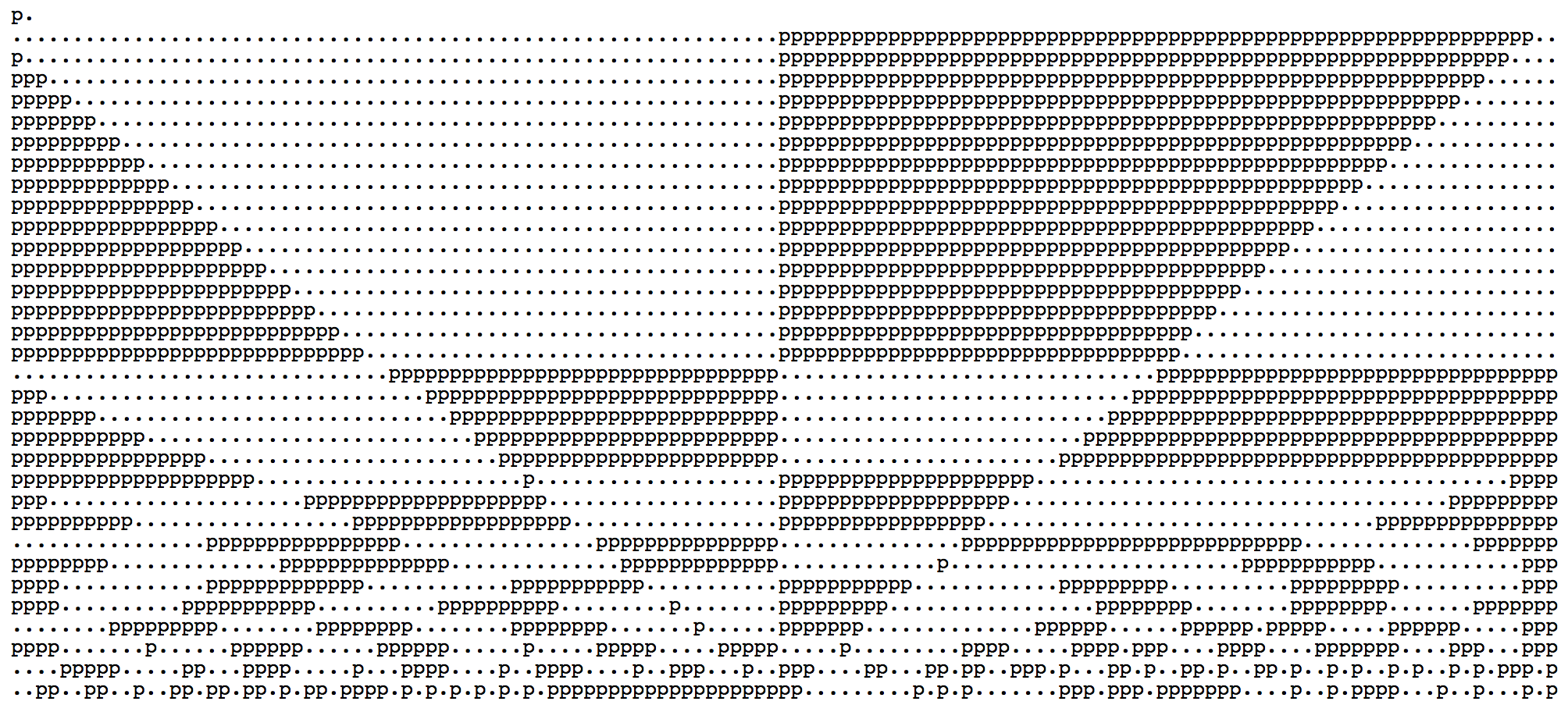The graph continues to loop indefinitely in a similar manner.

I hope this makes sense. I don’t think I would have ever been able to come-up with something like this on my own, but it was fun trying to understand it.